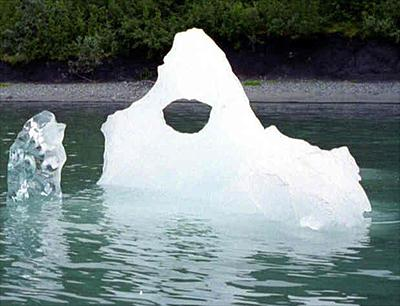#Hello, Guest!

### Lesson Plan## Regular Coke vs. Diet Coke: Which is Denser?

### Subject

Math, Science & Technology

### Description

This lesson is designed as an introduction to a density unit. Using products that students encounter every day, you can demonstrate the concepts of density, volume, and mass. By the end of this lesson, students will understand that, although cans of Regular and Diet Coke have the same volume, Regular Coke is denser because of the sugar content.

### Objectives

1. The students will define the terms "mass," "volume," and "density."
2. The students will understand the relationship between mass, volume, and density.
3. The students will observe the similarities and differences between the cans of Regular and Diet Coke.
4. The students will develop hypotheses about why a can of Regular Coke sinks and a can of Diet Coke floats.

• Coke
• Diet Coke
• Fish Tank
• Water
• Sugar
• NutraSweet

### Procedure

1. Review with students that volume is the amount of space taken up by a three-dimensional object.
2. Ask students how they would measure volume.
3. Tell students that we are now going to discuss density.
4. Explain that density is the ratio of mass to volume, and can be represented by the following formula:

Density = Mass/Volume

If two objects have the same volume, but one has more mass, the one with more mass is going to be denser.
5. Take out the two cans of Coke. Ask the students to make observations (similarities and differences) about the Regular Coke and Diet Coke. Write these observations on the board.
6. Drop the Regular Coke can into the tank of water and watch it sink.
7. Next, drop the Diet Coke into the water.
8. Ask one student to come check the cans to make sure that they are still sealed.
9. Ask the students why they think the Regular Coke sank and the Diet did not. Write these explanations on the board.
10. Show the students a beaker full of sugar (39 grams). Tell them that this is the amount of sugar in a can of Regular Coke.
11. Show the students a packet of NutraSweet and tell them that this is how much sugar is in a can of Diet Coke. Then have them try to answer the question again: Why did the Regular Coke sink, but the Diet Coke did not?
12. Students should develop hypotheses to explain the differences in density. Through discussion of volume, mass, and density, they should figure out that the large amount of sugar in the Regular Coke increases the mass, and thus makes it more dense than the Diet Coke.
13. Continue the lesson by giving more examples illustrating the concept of density. For example, you could use the real-world example of population density. Explain to students that a city is more dense compared to the country because there are more people in the city.